### Revenge of the Baby-SatHover over the thumbnail for a full-size version.

Author im_bad_at_N author:im_bad_at_n hobbes ibannart n-art rated 2010-01-15 2010-07-25 3 by 49 people. \$Revenge of the Baby-Sat#im_bad_at_N#n-art#00000000000000000000000000000000000000000000000000000000000000000000000000000000000000000000000000000000000000000000000000000000000000000000000000000000000000000000000000000000000000000000000000000000000000000000000000000000000000000000000000000000000000000000000000000000000000000000000000000000000000000000000000000000000000000000000000000000000000000000000000000000000000000000000000000000000000000000000000000000000000000000000000000000000000000000000000000000000000000000000000000000000000000000000000000000000000000000000000000000000000000000000000000000000000000000000000000000000000000000000000000000000000000000000000000000000000000000000000000000000000000000000000000000000000000000000000000000000000000|12^548,575!12^546,571!12^544,565!12^543,559!12^543,556!12^541,551!12^543,552!12^540,550!12^538,550!12^540,554!12^541,558!12^541,561!12^535,576!12^536,573!12^538,570!12^540,566!12^539,575!12^541,575!12^543,574!12^545,575!12^541,572!12^543,549!12^546,548!12^396,297!12^550,545!12^555,544!12^557,543!12^558,542!12^559,541!12^560,541!12^563,539!12^567,538!12^568,537!12^570,536!12^573,536!12^578,535!12^564,541!12^567,540!12^573,536!12^578,535!12^564,537!12^568,535!12^570,534!12^575,531!12^578,530!12^581,528!12^584,457!12^585,462!12^584,464!12^585,467!12^585,470!12^585,475!12^584,483!12^585,487!12^585,491!12^584,481!12^583,527!12^583,525!12^584,522!12^583,520!12^584,517!12^585,515!12^585,512!12^585,509!12^585,507!12^585,503!12^586,501!12^585,500!12^586,497!12^585,496!12^616,478!12^616,483!12^614,486!12^614,489!12^585,454!12^583,451!12^583,449!12^582,445!12^582,444!12^582,442!12^582,442!12^581,440!12^594,462!12^594,468!12^594,474!12^588,474!12^588,468!12^588,462!12^594,456!12^588,456!12^588,444!12^588,444!12^594,450!12^588,450!12^594,438!12^594,444!12^588,438!12^588,432!12^588,432!12^588,420!12^588,414!12^594,426!12^588,426!12^582,438!12^582,426!12^582,432!12^582,420!12^582,414!12^582,408!12^582,402!12^582,396!12^582,390!12^588,402!12^588,390!12^588,396!12^588,414!12^588,414!12^588,414!12^588,408!12^594,432!12^588,420!12^594,420!12^594,408!12^594,402!12^594,414!12^576,420!12^576,414!12^576,414!12^576,408!12^576,402!12^570,402!12^570,408!12^600,408!12^600,420!12^600,426!12^600,432!12^600,438!12^600,444!12^600,450!12^600,456!12^600,462!12^600,468!12^600,474!12^600,414!12^600,402!12^600,396!12^606,408!12^606,414!12^606,420!12^606,432!12^606,426!12^606,438!12^606,444!12^606,450!12^606,456!12^606,462!12^606,468!12^606,474!12^612,420!12^612,420!12^612,432!12^612,438!12^612,444!12^612,450!12^612,456!12^612,462!12^612,468!12^612,474!12^612,426!12^612,414!12^588,480!12^594,480!12^606,480!12^606,480!12^612,480!12^600,480!12^588,486!12^600,492!12^594,486!12^600,486!12^606,486!12^612,486!12^606,498!12^606,492!12^612,492!12^612,504!12^606,504!12^600,504!12^594,504!12^588,504!12^594,498!12^600,498!12^594,498!12^594,492!12^588,492!12^588,498!12^612,498!12^606,510!12^600,510!12^594,510!12^594,510!12^588,510!12^588,516!12^594,516!12^600,516!12^606,516!12^594,522!12^588,522!12^588,528!12^600,522!12^612,516!12^618,516!12^600,522!12^606,522!12^579,435!12^578,431!12^578,427!12^577,426!12^576,424!12^574,422!12^573,419!12^563,399!12^564,403!12^566,404!12^568,406!12^569,409!12^571,412!12^573,416!12^566,399!12^568,399!12^571,400!12^573,399!12^576,398!12^581,394!12^580,393!12^580,391!12^580,389!12^580,388!12^591,397!12^594,398!12^596,398!12^603,401!12^605,402!12^607,405!12^609,408!12^610,409!12^612,412!12^613,414!12^613,415!12^614,418!12^616,421!12^617,425!12^616,429!12^616,433!12^616,437!12^616,443!12^617,447!12^616,450!12^615,453!12^615,456!12^615,460!12^615,462!12^615,468!12^614,470!12^614,474!12^616,461!12^615,454!12^616,446!12^617,442!12^616,438!12^618,434!12^617,433!12^616,426!12^609,512!12^610,508!12^611,508!12^584,532!12^583,532!12^588,531!12^591,525!12^590,528!12^592,527!12^596,526!12^599,524!12^602,523!12^612,519!12^616,516!12^618,516!12^621,514!12^624,513!12^628,512!12^627,511!12^630,511!12^632,509!12^634,509!12^636,508!12^640,508!12^642,507!12^645,505!12^649,505!12^653,503!12^656,503!12^659,502!12^663,502!12^662,500!12^665,499!12^667,498!12^669,498!12^671,498!12^675,498!12^674,497!12^675,495!12^679,494!12^681,494!12^684,494!12^615,513!12^616,514!12^702,426!12^702,432!12^702,444!12^702,438!12^702,450!12^702,456!12^702,462!12^702,468!12^696,468!12^696,462!12^696,474!12^696,480!12^690,480!12^690,492!12^690,486!12^696,486!12^696,474!12^702,474!12^708,468!12^708,462!12^708,456!12^708,450!12^708,444!12^708,438!12^708,432!12^708,426!12^708,420!12^708,414!12^708,408!12^708,402!12^708,396!12^708,390!12^714,396!12^714,402!12^714,408!12^714,414!12^714,420!12^714,432!12^714,438!12^714,420!12^714,432!12^714,444!12^714,426!12^714,450!12^714,456!12^720,444!12^720,432!12^720,426!12^720,420!12^720,414!12^720,414!12^720,408!12^720,402!12^720,396!12^714,390!12^702,420!12^702,414!12^702,408!12^696,408!12^696,402!12^696,402!12^696,396!12^690,390!12^696,390!12^684,492!12^686,488!12^686,486!12^687,482!12^690,478!12^693,473!12^694,469!12^694,466!12^695,463!12^696,461!12^695,456!12^696,452!12^696,450!12^696,448!12^696,447!12^697,444!12^698,440!12^698,440!12^699,438!12^699,436!12^699,433!12^699,428!12^696,423!12^694,411!12^695,413!12^696,416!12^696,420!12^697,425!12^698,431!12^697,435!12^685,387!12^688,389!12^690,390!12^691,394!12^692,397!12^694,401!12^694,404!12^695,410!12^690,384!12^692,386!12^693,386!12^695,386!12^697,386!12^700,386!12^702,386!12^704,388!12^709,389!12^699,395!12^705,395!12^717,390!12^721,391!12^723,392!12^724,394!12^725,398!12^725,402!12^723,408!12^723,412!12^723,417!12^722,422!12^722,426!12^720,431!12^720,434!12^718,439!12^718,442!12^717,448!12^716,452!12^715,454!12^713,456!12^713,460!12^712,463!12^711,466!12^709,470!12^707,473!12^704,476!12^702,479!12^701,481!12^697,485!12^694,488!12^723,427!12^724,417!12^726,411!12^697,489!12^703,488!12^707,486!12^710,486!12^712,485!12^716,483!12^717,483!12^719,483!12^722,482!12^723,481!12^724,480!12^725,480!12^727,479!12^729,478!12^732,478!12^734,477!12^736,477!12^737,477!12^740,475!12^746,474!12^748,474!12^750,473!12^752,472!12^755,471!12^757,471!12^759,469!12^761,468!12^764,468!12^765,468!12^767,468!12^768,467!12^769,465!12^687,495!12^768,407!12^766,406!12^765,405!12^763,405!12^760,404!12^759,403!12^757,402!12^754,402!12^752,401!12^750,400!12^749,400!12^746,398!12^745,398!12^742,397!12^726,392!12^727,393!12^728,393!12^729,393!12^733,395!12^733,395!12^736,396!12^739,397!12^696,432!12^696,429!12^755,400!12^757,399!12^759,397!12^760,396!12^761,393!12^763,390!12^763,387!12^764,384!12^766,383!12^767,380!12^768,377!12^768,374!12^768,372!12^770,365!12^769,368!12^765,400!12^766,397!12^767,390!12^768,395!12^766,398!12^768,403!12^768,394!12^768,385!12^501,545!12^504,547!12^503,550!12^505,552!12^505,552!12^507,555!12^507,556!12^508,557!12^509,562!12^511,565!12^511,567!12^512,570!12^513,572!12^515,575!12^515,576!12^503,544!12^505,544!12^506,543!12^507,541!12^508,541!12^508,541!12^511,538!12^512,537!12^513,534!12^515,532!12^516,529!12^516,527!12^498,541!12^502,540!12^506,538!12^509,536!12^511,541!12^511,541!12^511,542!12^508,543!12^482,519!12^482,520!12^484,522!12^486,524!12^487,525!12^488,527!12^490,529!12^491,531!12^492,533!12^495,535!12^496,537!12^498,539!12^499,541!12^485,522!12^488,523!12^489,524!12^487,524!12^486,522!12^485,519!12^487,519!12^488,520!12^491,518!12^488,519!12^490,519!12^495,520!12^497,519!12^498,519!12^499,519!12^501,519!12^504,519!12^504,519!12^506,519!12^507,519!12^504,517!12^504,516!12^471,491!12^473,493!12^474,494!12^476,495!12^479,497!12^480,497!12^482,499!12^484,500!12^486,501!12^488,503!12^490,504!12^491,504!12^493,505!12^495,506!12^496,507!12^498,508!12^499,509!12^500,511!12^501,513!12^502,515!12^503,516!12^470,488!12^469,486!12^472,486!12^474,486!12^478,487!12^482,485!12^484,485!12^486,485!12^488,485!12^490,484!12^492,483!12^493,483!12^496,483!12^499,483!12^500,483!12^502,483!12^504,483!12^460,467!12^462,467!12^464,468!12^468,469!12^470,470!12^472,471!12^475,471!12^478,472!12^479,473!12^480,474!12^483,475!12^486,476!12^487,476!12^489,477!12^493,479!12^497,481!12^497,481!12^503,483!12^455,462!12^461,462!12^465,462!12^454,462!12^457,460!12^458,459!12^459,458!12^460,457!12^462,455!12^462,454!12^463,450!12^464,450!12^465,447!12^466,445!12^466,443!12^466,440!12^466,440!12^466,446!12^467,445!12^470,445!12^471,444!12^441,447!12^444,448!12^447,448!12^450,447!12^452,446!12^454,446!12^456,446!12^459,445!12^460,444!12^463,444!12^466,444!12^451,450!12^456,448!12^459,444!12^462,444!12^474,414!12^474,420!12^480,420!12^480,426!12^486,426!12^486,432!12^492,432!12^492,432!12^492,438!12^498,438!12^498,444!12^504,444!12^504,450!12^492,426!12^498,432!12^486,420!12^486,414!12^486,414!12^480,414!12^468,420!12^468,414!12^468,408!12^462,408!12^462,414!12^474,408!12^480,408!12^480,408!12^486,408!12^486,402!12^513,479!12^513,476!12^512,474!12^511,471!12^510,467!12^510,466!12^509,464!12^507,459!12^507,456!12^505,452!12^508,460!12^503,457!12^483,433!12^485,435!12^488,438!12^490,440!12^492,442!12^495,445!12^496,448!12^499,451!12^501,454!12^504,459!12^492,416!12^493,419!12^494,422!12^495,425!12^496,427!12^497,429!12^499,433!12^500,437!12^501,440!12^503,444!12^505,448!12^506,451!12^489,420!12^489,415!12^488,413!12^471,424!12^474,426!12^476,428!12^478,430!12^481,432!12^436,409!12^438,409!12^441,410!12^444,411!12^447,411!12^449,412!12^452,413!12^454,414!12^458,415!12^461,418!12^463,420!12^466,421!12^469,422!12^436,401!12^440,403!12^442,404!12^445,409!12^450,410!12^452,411!12^455,411!12^459,409!12^448,388!12^450,392!12^451,394!12^452,396!12^453,398!12^456,401!12^458,403!12^461,405!12^464,406!12^462,403!12^467,404!12^469,404!12^473,404!12^475,404!12^476,404!12^480,404!12^471,403!12^474,402!12^477,402!12^480,402!12^484,402!12^486,402!12^447,384!12^448,383!12^449,382!12^450,382!12^453,382!12^454,382!12^457,382!12^459,384!12^461,385!12^464,386!12^467,388!12^468,389!12^470,390!12^472,391!12^475,392!12^477,393!12^479,394!12^481,395!12^483,397!12^484,397!12^485,397!12^487,397!12^489,397!12^490,397!12^466,363!12^467,364!12^468,365!12^469,368!12^470,369!12^473,375!12^474,376!12^477,379!12^479,381!12^480,384!12^482,385!12^485,388!12^486,389!12^489,393!12^491,393!12^471,373!12^493,400!12^495,400!12^494,399!12^495,397!12^495,397!12^491,391!12^489,390!12^489,387!12^488,385!12^489,384!12^490,381!12^490,381!12^491,380!12^489,377!12^492,375!12^494,373!12^494,370!12^495,367!12^495,365!12^497,362!12^498,358!12^499,356!12^501,355!12^501,352!12^502,349!12^503,348!12^503,345!12^504,342!12^504,341!12^504,339!12^503,337!12^502,334!12^502,328!12^503,326!12^503,325!12^503,322!12^504,319!12^499,399!12^501,399!12^503,399!12^504,399!12^505,400!12^506,400!12^507,401!12^508,402!12^490,402!12^494,404!12^496,403!12^498,403!12^501,403!12^504,403!12^506,402!12^508,403!12^510,406!12^511,406!12^514,407!12^518,408!12^519,409!12^523,409!12^523,410!12^524,410!12^526,411!12^529,411!12^532,413!12^535,414!12^538,414!12^541,414!12^543,414!12^545,411!12^547,408!12^541,412!12^544,410!12^547,409!12^550,407!12^551,406!12^554,405!12^555,404!12^558,402!12^559,399!12^560,398!12^560,398!12^542,387!12^543,386!12^546,384!12^548,383!12^549,381!12^550,380!12^550,378!12^553,379!12^554,381!12^557,382!12^560,382!12^564,383!12^567,383!12^570,385!12^573,386!12^576,386!12^564,387!12^563,389!12^563,392!12^562,394!12^561,396!12^558,399!12^581,388!12^583,389!12^586,388!12^589,388!12^593,388!12^597,387!12^600,386!12^602,386!12^580,387!12^577,387!12^584,389!12^597,396!12^593,395!12^600,395!12^603,396!12^600,395!12^604,393!12^607,392!12^608,392!12^611,391!12^613,391!12^618,391!12^620,390!12^623,389!12^627,389!12^628,389!12^630,388!12^631,388!12^634,388!12^602,384!12^609,384!12^611,383!12^605,383!12^610,382!12^614,381!12^615,380!12^617,380!12^619,379!12^621,378!12^623,377!12^623,375!12^629,374!12^630,374!12^632,373!12^634,373!12^636,372!12^637,371!12^637,370!12^640,370!12^641,370!12^643,369!12^644,369!12^645,369!12^632,376!12^634,374!12^637,374!12^638,374!12^639,374!12^641,374!12^642,375!12^643,376!12^645,377!12^646,377!12^647,377!12^649,377!12^660,378!12^672,378!12^666,378!12^654,378!12^672,372!12^672,366!12^672,360!12^666,372!12^684,342!12^678,348!12^678,354!12^678,354!12^678,360!12^678,366!12^678,342!12^678,336!12^678,330!12^678,324!12^678,318!12^678,312!12^684,312!12^684,318!12^684,318!12^684,330!12^684,336!12^684,324!12^678,306!12^678,300!12^678,294!12^678,294!12^678,288!12^672,288!12^672,294!12^684,294!12^684,288!12^684,288!12^696,288!12^702,288!12^702,288!12^690,288!12^708,288!12^714,288!12^720,288!12^690,300!12^690,306!12^690,312!12^690,318!12^690,324!12^690,336!12^684,342!12^690,330!12^684,306!12^684,300!12^696,306!12^696,312!12^696,318!12^690,330!12^690,330!12^696,336!12^696,330!12^696,324!12^690,342!12^696,342!12^690,348!12^684,348!12^684,354!12^690,354!12^666,288!12^660,288!12^678,378!12^678,372!12^654,384!12^660,384!12^666,384!12^672,384!12^726,288!12^762,294!12^768,294!12^762,300!12^762,300!12^768,300!12^768,306!12^768,312!12^768,318!12^756,294!12^757,290!12^760,291!12^762,291!12^765,291!12^758,299!12^760,301!12^763,304!12^764,305!12^765,310!12^767,314!12^767,315!12^766,321!12^769,329!12^770,334!12^770,338!12^770,330!12^768,323!12^731,293!12^733,292!12^736,291!12^738,291!12^741,292!12^743,292!12^745,293!12^748,294!12^750,296!12^754,298!12^757,300!12^705,291!12^707,291!12^711,291!12^715,291!12^718,291!12^721,291!12^725,291!12^728,291!12^732,292!12^686,293!12^687,293!12^698,340!12^700,337!12^700,336!12^703,333!12^703,330!12^704,329!12^703,328!12^702,325!12^702,324!12^702,321!12^701,318!12^701,317!12^700,316!12^699,315!12^699,311!12^698,310!12^696,306!12^692,301!12^690,299!12^700,313!12^697,308!12^700,324!12^698,330!12^693,356!12^693,355!12^693,354!12^694,350!12^695,349!12^696,345!12^683,381!12^684,378!12^685,376!12^685,376!12^686,371!12^688,369!12^689,364!12^689,363!12^691,359!12^680,367!12^681,364!12^684,359!12^687,355!12^654,376!12^656,374!12^659,373!12^660,372!12^661,372!12^664,368!12^664,367!12^666,363!12^666,362!12^668,361!12^670,357!12^671,354!12^671,352!12^671,351!12^672,348!12^673,346!12^673,344!12^674,343!12^674,340!12^674,339!12^674,338!12^675,335!12^671,297!12^671,298!12^673,300!12^673,300!12^674,302!12^675,306!12^675,308!12^676,310!12^676,312!12^676,316!12^674,319!12^674,326!12^674,329!12^673,333!12^673,335!12^668,292!12^667,295!12^664,291!12^663,290!12^727,287!12^729,288!12^732,288!12^736,288!12^738,288!12^742,289!12^746,289!12^749,289!12^753,289!12^649,286!12^650,286!12^655,286!12^659,286!12^664,287!12^666,287!12^672,287!12^678,287!12^683,287!12^648,294!12^647,292!12^646,287!12^645,286!12^643,284!12^652,287!12^657,289!12^678,382!12^678,385!12^679,367!12^685,363!12^682,368!12^636,388!12^635,389!12^639,388!12^641,386!12^644,386!12^648,385!12^651,384!12^653,382!12^605,261!12^610,263!12^613,263!12^615,263!12^617,265!12^619,268!12^621,269!12^624,272!12^626,273!12^627,276!12^630,277!12^634,279!12^637,281!12^640,282!12^644,284!12^602,237!12^604,239!12^605,241!12^606,243!12^607,246!12^610,250!12^612,253!12^615,257!12^616,260!12^611,257!12^608,254!12^608,251!12^606,245!12^604,240!12^578,216!12^580,219!12^581,221!12^583,224!12^585,226!12^587,226!12^589,228!12^591,229!12^593,231!12^595,232!12^599,235!12^602,237!12^581,178!12^579,182!12^578,185!12^578,189!12^578,192!12^577,195!12^576,198!12^576,202!12^575,206!12^576,210!12^577,213!12^578,214!12^579,210!12^580,207!12^579,201!12^578,196!12^579,193!12^580,188!12^581,185!12^582,181!12^582,179!12^579,177!12^579,176!12^579,173!12^689,290!12^692,288!12^693,288!12^696,288!12^535,177!12^535,174!12^538,174!12^538,174!12^541,172!12^542,171!12^545,171!12^547,170!12^548,168!12^550,167!12^552,167!12^556,167!12^558,166!12^561,166!12^563,166!12^565,167!12^566,167!12^569,167!12^571,168!12^573,168!12^575,168!12^577,169!12^579,171!12^581,174!12^580,172!12^577,170!12^526,194!12^528,189!12^530,188!12^532,184!12^534,181!12^536,177!12^529,226!12^528,223!12^527,219!12^527,217!12^526,213!12^526,210!12^526,208!12^526,205!12^526,201!12^526,197!12^532,230!12^536,231!12^537,232!12^540,234!12^544,234!12^546,234!12^550,235!12^552,236!12^554,237!12^558,238!12^560,239!12^563,241!12^566,243!12^569,246!12^505,235!12^507,235!12^510,234!12^513,233!12^517,233!12^520,233!12^524,232!12^529,231!12^532,231!12^518,232!12^525,230!12^467,237!12^470,236!12^473,235!12^476,235!12^478,235!12^482,235!12^485,235!12^490,236!12^495,236!12^498,236!12^501,236!12^503,235!12^488,215!12^493,219!12^495,219!12^500,218!12^504,217!12^506,217!12^509,217!12^513,215!12^485,215!12^489,210!12^492,208!12^493,207!12^494,203!12^495,200!12^495,200!12^495,198!12^497,196!12^501,192!12^502,191!12^504,189!12^506,187!12^507,186!12^509,183!12^511,181!12^513,178!12^515,176!12^456,205!12^459,204!12^463,202!12^466,197!12^468,195!12^471,193!12^471,193!12^472,192!12^478,190!12^478,190!12^479,190!12^484,188!12^489,185!12^492,182!12^494,181!12^497,180!12^501,177!12^503,176!12^507,175!12^511,172!12^512,172!12^517,168!12^454,157!12^455,158!12^455,160!12^453,164!12^452,170!12^452,173!12^452,177!12^451,181!12^451,184!12^452,187!12^452,190!12^451,195!12^451,200!12^452,204!12^452,206!12^432,204!12^426,204!12^432,198!12^426,198!12^435,204!12^438,198!12^436,197!12^438,194!12^438,192!12^438,190!12^440,187!12^441,184!12^442,182!12^444,181!12^445,179!12^445,176!12^446,175!12^448,171!12^449,169!12^450,166!12^451,164!12^453,160!12^423,205!12^421,203!12^419,202!12^418,202!12^423,199!12^424,196!12^426,195!12^427,194!12^430,206!12^402,192!12^408,192!12^402,198!12^401,203!12^402,201!12^403,199!12^405,198!12^408,197!12^410,195!12^413,193!12^414,192!12^407,190!12^405,190!12^403,187!12^381,198!12^382,197!12^383,196!12^387,193!12^390,191!12^394,189!12^384,193!12^383,195!12^382,201!12^382,185!12^385,186!12^385,173!12^388,176!12^389,180!12^390,185!12^397,171!12^398,175!12^399,178!12^400,183!12^401,187!12^405,161!12^404,163!12^406,164!12^407,166!12^409,167!12^410,169!12^410,171!12^412,173!12^412,174!12^413,176!12^414,178!12^416,179!12^418,182!12^419,184!12^421,187!12^422,189!12^425,191!12^368,147!12^371,147!12^375,147!12^380,148!12^383,148!12^384,147!12^386,145!12^389,144!12^402,150!12^396,150!12^390,150!12^402,156!12^402,144!12^396,144!12^408,144!12^408,150!12^414,144!12^414,132!12^414,132!12^414,114!12^414,108!12^414,114!12^414,126!12^414,120!12^408,138!12^414,138!12^420,96!12^420,102!12^420,108!12^420,114!12^420,120!12^420,120!12^420,126!12^420,132!12^420,138!12^426,102!12^426,108!12^426,108!12^426,120!12^426,114!12^426,126!12^414,102!12^414,90!12^414,90!12^414,84!12^414,90!12^414,96!12^420,90!12^408,102!12^408,96!12^408,96!12^402,96!12^396,96!12^390,96!12^342,102!12^348,102!12^348,102!12^360,120!12^366,126!12^366,120!12^372,120!12^372,114!12^378,108!12^384,102!12^390,90!12^402,90!12^402,90!12^408,90!12^402,84!12^408,84!12^396,78!12^402,78!12^402,78!12^330,114!12^383,143!12^386,144!12^389,144!12^394,142!12^395,142!12^397,141!12^399,139!12^401,138!12^403,136!12^405,133!12^407,132!12^409,127!12^410,126!12^410,124!12^410,121!12^409,119!12^408,116!12^408,114!12^408,111!12^407,110!12^405,109!12^403,105!12^401,104!12^399,104!12^397,104!12^394,104!12^392,105!12^389,108!12^387,109!12^384,111!12^383,112!12^383,113!12^381,113!12^380,114!12^380,116!12^378,118!12^377,121!12^376,122!12^374,125!12^373,126!12^372,128!12^371,129!12^371,129!12^370,130!12^391,105!12^391,103!12^384,105!12^379,111!12^403,158!12^401,156!12^399,154!12^397,152!12^394,152!12^390,152!12^387,152!12^404,156!12^406,155!12^407,154!12^409,153!12^410,152!12^412,151!12^414,148!12^415,146!12^417,145!12^418,144!12^419,142!12^421,141!12^430,102!12^431,103!12^429,106!12^429,108!12^429,110!12^428,113!12^427,116!12^427,119!12^426,123!12^425,125!12^424,128!12^422,131!12^418,145!12^420,141!12^422,138!12^424,134!12^425,130!12^426,127!12^428,120!12^428,119!12^428,114!12^428,111!12^429,107!12^429,106!12^415,150!12^417,82!12^419,83!12^420,85!12^420,86!12^422,89!12^423,90!12^424,92!12^425,94!12^427,97!12^430,98!12^432,105!12^430,99!12^429,97!12^428,96!12^427,94!12^399,75!12^401,76!12^404,76!12^405,76!12^408,79!12^412,79!12^415,80!12^369,77!12^371,77!12^374,77!12^377,76!12^380,76!12^382,75!12^386,75!12^389,75!12^393,74!12^343,100!12^348,99!12^352,94!12^354,92!12^357,90!12^358,88!12^360,85!12^362,83!12^363,81!12^366,80!12^336,112!12^338,108!12^340,105!12^343,101!12^318,113!12^320,113!12^323,113!12^327,114!12^329,117!12^330,119!12^333,120!12^335,120!12^338,122!12^340,125!12^342,126!12^338,113!12^358,122!12^363,125!12^368,128!12^370,130!12^380,231!12^382,228!12^394,214!12^397,212!12^400,214!12^402,215!12^405,217!12^408,218!12^410,218!12^414,218!12^416,219!12^419,220!12^421,221!12^423,222!12^425,224!12^427,225!12^392,232!12^393,231!12^397,229!12^400,229!12^401,229!12^405,227!12^406,226!12^405,228!12^409,226!12^412,226!12^415,226!12^417,225!12^420,225!12^422,225!12^424,224!12^426,225!12^431,225!12^432,225!12^433,225!12^435,225!12^436,224!12^438,224!12^440,225!12^443,225!12^446,225!12^447,225!12^448,225!12^455,225!12^450,224!12^453,224!12^459,225!12^461,225!12^464,226!12^468,226!12^470,228!12^472,229!12^475,230!12^476,230!12^479,231!12^479,232!12^480,232!12^443,243!12^445,243!12^450,242!12^453,240!12^456,240!12^459,239!12^460,238!12^463,237!12^430,123!12^429,122!12^429,120!12^431,118!12^432,117!12^431,115!12^432,112!12^432,111!12^432,109!12^284,115!12^285,116!12^289,114!12^290,113!12^292,112!12^294,111!12^296,111!12^299,111!12^301,111!12^304,111!12^308,111!12^312,112!12^315,112!12^285,105!12^284,107!12^284,108!12^283,111!12^282,114!12^280,116!12^279,117!12^277,118!12^281,118!12^284,118!12^239,127!12^239,127!12^243,124!12^244,123!12^246,121!12^249,119!12^250,118!12^251,118!12^253,117!12^255,115!12^257,114!12^259,114!12^260,113!12^261,111!12^264,110!12^266,109!12^269,107!12^270,106!12^272,105!12^274,103!12^276,102!12^277,100!12^279,97!12^249,93!12^246,96!12^245,97!12^245,99!12^245,102!12^244,105!12^243,108!12^242,111!12^240,114!12^239,118!12^239,122!12^238,124!12^206,137!12^209,135!12^210,134!12^213,132!12^215,129!12^218,127!12^219,126!12^220,125!12^221,123!12^223,120!12^225,117!12^226,116!12^227,115!12^228,113!12^230,111!12^233,108!12^235,107!12^238,104!12^240,101!12^243,99!12^244,96!12^246,270!12^252,270!12^264,270!12^258,270!12^276,270!12^282,270!12^270,270!12^288,270!12^294,264!12^282,264!12^276,264!12^270,264!12^264,264!12^252,264!12^246,258!12^258,264!12^246,264!12^258,258!12^264,258!12^270,258!12^276,258!12^276,258!12^282,258!12^288,258!12^288,264!12^294,258!12^294,252!12^294,246!12^282,246!12^276,252!12^264,252!12^258,252!12^258,252!12^252,258!12^252,258!12^246,252!12^252,252!12^252,252!12^252,246!12^264,246!12^264,246!12^270,246!12^276,246!12^270,252!12^258,246!12^264,240!12^270,240!12^270,240!12^276,240!12^282,240!12^288,240!12^288,246!12^282,252!12^282,252!12^288,252!12^294,240!12^300,234!12^300,234!12^300,240!12^300,246!12^300,252!12^300,258!12^300,264!12^294,276!12^300,270!12^294,270!12^246,276!12^252,276!12^258,276!12^258,276!12^264,276!12^270,276!12^270,276!12^276,270!12^282,270!12^282,276!12^276,276!12^288,276!12^246,282!12^252,282!12^258,282!12^264,282!12^270,282!12^276,282!12^282,282!12^288,282!12^294,282!12^240,282!12^240,276!12^240,276!12^240,270!12^240,264!12^246,258!12^246,258!12^240,258!12^240,258!12^240,252!12^240,246!12^240,240!12^246,240!12^246,246!12^246,246!12^252,240!12^258,240!12^258,240!12^234,252!12^234,258!12^234,264!12^234,264!12^234,276!12^240,270!12^234,270!12^228,270!12^228,264!12^228,264!12^228,258!12^228,276!12^252,234!12^258,234!12^264,234!12^270,234!12^276,234!12^282,234!12^282,234!12^288,234!12^294,234!12^264,228!12^270,228!12^276,228!12^282,228!12^288,228!12^294,228!12^300,228!12^276,222!12^282,222!12^288,222!12^294,222!12^294,222!12^288,216!12^294,216!12^300,216!12^300,216!12^306,222!12^306,216!12^300,222!12^306,228!12^306,234!12^306,240!12^306,240!12^306,246!12^306,252!12^306,258!12^312,252!12^312,246!12^312,240!12^312,234!12^312,234!12^312,228!12^312,222!12^312,222!12^318,228!12^318,234!12^318,240!12^306,264!12^318,246!12^318,222!12^306,282!12^300,282!12^300,276!12^312,258!12^246,294!12^252,294!12^258,294!12^264,294!12^270,294!12^276,294!12^282,294!12^252,288!12^258,288!12^264,288!12^264,288!12^270,288!12^276,288!12^282,288!12^288,288!12^288,288!12^294,288!12^234,294!12^240,294!12^234,288!12^240,288!12^246,288!12^228,294!12^228,288!12^222,288!12^234,281!12^232,280!12^231,279!12^229,278!12^227,277!12^225,275!12^225,274!12^223,272!12^223,270!12^223,268!12^224,264!12^225,260!12^226,260!12^226,259!12^226,256!12^228,252!12^229,252!12^232,250!12^232,250!12^233,248!12^234,246!12^235,244!12^236,243!12^238,242!12^240,240!12^243,238!12^243,236!12^244,235!12^246,234!12^249,232!12^251,231!12^253,229!12^255,228!12^257,227!12^259,226!12^260,226!12^263,225!12^266,223!12^268,223!12^268,223!12^270,222!12^271,220!12^273,219!12^276,218!12^277,217!12^280,216!12^282,216!12^284,215!12^287,215!12^289,215!12^292,214!12^296,214!12^299,214!12^301,214!12^303,215!12^307,216!12^308,216!12^310,216!12^312,217!12^314,219!12^318,221!12^318,221!12^320,224!12^320,227!12^320,230!12^318,233!12^317,235!12^317,240!12^304,272!12^306,269!12^308,267!12^310,264!12^312,261!12^313,260!12^314,259!12^314,257!12^316,256!12^316,255!12^317,253!12^317,251!12^317,250!12^318,248!12^319,247!12^320,244!12^320,243!12^320,240!12^320,238!12^321,234!12^321,230!12^320,227!12^318,276!12^312,276!12^324,270!12^318,270!12^330,264!12^330,258!12^305,280!12^308,279!12^310,279!12^313,276!12^314,275!12^317,273!12^318,272!12^319,271!12^322,268!12^323,267!12^324,266!12^325,264!12^326,261!12^329,259!12^330,256!12^304,284!12^306,284!12^310,283!12^312,281!12^315,279!12^316,279!12^318,277!12^318,277!12^320,276!12^323,273!12^324,273!12^326,271!12^328,270!12^329,268!12^331,265!12^332,263!12^336,219!12^337,221!12^337,224!12^336,226!12^336,229!12^335,232!12^335,236!12^335,239!12^336,243!12^336,245!12^334,249!12^334,252!12^333,255!12^332,259!12^334,251!12^336,245!12^339,224!12^338,226!12^337,234!12^336,239!12^320,203!12^323,204!12^324,205!12^326,207!12^329,208!12^330,210!12^333,212!12^335,213!12^337,215!12^325,209!12^329,211!12^331,213!12^333,215!12^334,218!12^335,223!12^335,225!12^282,198!12^288,198!12^294,198!12^300,198!12^306,198!12^312,198!12^276,198!12^270,204!12^282,204!12^264,204!12^276,204!12^283,204!12^285,203!12^286,203!12^289,203!12^292,202!12^293,202!12^296,202!12^299,202!12^301,202!12^303,202!12^306,202!12^308,202!12^310,202!12^314,202!12^317,203!12^315,197!12^318,196!12^321,196!12^321,196!12^324,195!12^326,194!12^330,194!12^333,194!12^337,194!12^341,194!12^344,194!12^347,194!12^350,195!12^355,195!12^357,194!12^303,194!12^306,194!12^313,191!12^317,189!12^319,188!12^322,188!12^324,187!12^328,185!12^331,184!12^335,184!12^336,183!12^337,181!12^340,180!12^291,192!12^297,193!12^301,193!12^308,193!12^280,197!12^281,191!12^281,190!12^284,187!12^287,184!12^288,182!12^289,181!12^292,178!12^295,177!12^295,177!12^297,175!12^299,173!12^300,171!12^303,169!12^304,169!12^307,167!12^310,165!12^313,163!12^315,162!12^317,161!12^319,160!12^321,159!12^324,158!12^325,157!12^327,157!12^329,157!12^331,157!12^332,157!12^334,156!12^336,156!12^338,156!12^340,156!12^343,156!12^345,156!12^348,157!12^351,158!12^353,159!12^354,160!12^358,162!12^359,163!12^360,163!12^344,148!12^347,149!12^349,150!12^350,152!12^352,154!12^354,156!12^355,157!12^357,158!12^360,161!12^361,162!12^363,165!12^273,199!12^273,195!12^271,194!12^270,192!12^270,189!12^270,186!12^270,183!12^271,181!12^271,178!12^271,177!12^271,175!12^271,173!12^272,169!12^274,167!12^274,165!12^274,163!12^277,159!12^279,156!12^279,155!12^282,152!12^282,152!12^283,150!12^285,148!12^245,213!12^249,212!12^251,211!12^252,210!12^254,208!12^256,207!12^259,206!12^262,206!12^265,205!12^248,206!12^257,204!12^259,203!12^262,201!12^265,199!12^268,199!12^254,183!12^256,186!12^257,189!12^258,193!12^259,195!12^260,197!12^231,168!12^233,169!12^237,172!12^239,173!12^240,175!12^241,177!12^243,179!12^244,181!12^245,183!12^246,185!12^248,188!12^249,190!12^250,194!12^251,196!12^253,200!12^253,201!12^185,172!12^187,172!12^187,171!12^188,170!12^190,169!12^192,167!12^193,167!12^196,166!12^198,165!12^198,165!12^201,164!12^203,164!12^207,163!12^209,163!12^211,163!12^213,163!12^216,163!12^217,164!12^220,164!12^222,165!12^225,165!12^227,166!12^227,166!12^185,173!12^182,174!12^180,178!12^178,180!12^176,182!12^175,183!12^174,184!12^174,187!12^171,191!12^170,192!12^169,196!12^168,199!12^168,200!12^167,203!12^167,205!12^166,207!12^165,211!12^164,213!12^164,217!12^162,220!12^163,223!12^164,225!12^164,226!12^165,230!12^165,234!12^165,237!12^168,240!12^169,242!12^171,245!12^172,249!12^175,254!12^177,257!12^179,260!12^181,261!12^165,267!12^167,266!12^173,264!12^176,263!12^178,264!12^175,268!12^175,272!12^183,258!12^183,258!12^186,256!12^189,254!12^191,251!12^194,247!12^196,246!12^198,243!12^200,242!12^204,241!12^192,267!12^194,266!12^198,263!12^200,263!12^210,258!12^210,258!12^210,264!12^210,270!12^210,270!12^210,276!12^210,282!12^216,282!12^210,252!12^210,246!12^210,240!12^216,240!12^234,216!12^240,216!12^228,216!12^228,222!12^222,234!12^284,292!12^286,292!12^287,292!12^290,291!12^294,290!12^298,290!12^301,288!12^243,297!12^249,298!12^253,298!12^258,299!12^263,298!12^268,297!12^273,296!12^213,283!12^215,285!12^217,287!12^220,290!12^224,293!12^229,294!12^235,296!12^241,298!12^209,248!12^208,253!12^207,254!12^207,258!12^206,262!12^206,266!12^206,270!12^208,274!12^210,277!12^212,251!12^214,247!12^214,245!12^216,241!12^218,238!12^219,235!12^222,230!12^224,229!12^227,227!12^228,225!12^231,221!12^232,221!12^235,220!12^212,240!12^214,235!12^218,232!12^220,230!12^233,193!12^235,196!12^236,199!12^238,202!12^241,205!12^243,208!12^230,192!12^228,194!12^226,195!12^225,197!12^224,199!12^223,200!12^223,203!12^223,206!12^223,208!12^224,211!12^226,216!12^228,219!12^229,221!12^227,215!12^228,213!12^230,214!12^232,214!12^234,214!12^236,213!12^231,199!12^233,202!12^234,205!12^236,208!12^239,211!12^182,151!12^184,150!12^186,146!12^187,142!12^188,141!12^190,137!12^191,134!12^193,131!12^195,129!12^196,126!12^198,125!12^200,122!12^201,121!12^203,118!12^204,114!12^206,112!12^207,110!12^209,108!12^211,106!12^209,108!12^209,109!12^207,118!12^207,123!12^207,128!12^207,130!12^144,211!12^144,205!12^145,200!12^145,194!12^146,191!12^147,186!12^147,183!12^149,179!12^151,176!12^152,173!12^155,171!12^157,169!12^158,167!12^161,165!12^163,163!12^165,162!12^167,161!12^170,160!12^175,158!12^178,156!12^182,153!12^182,152!12^130,207!12^131,206!12^135,202!12^136,198!12^137,195!12^140,191!12^141,189!12^144,186!12^366,85!12^370,83!12^373,82!12^376,81!12^378,80!12^343,108!12^346,104!12^376,89!12^379,89!12^382,88!12^386,87!12^389,87!12^394,87!12^397,87!12^394,85!12^365,103!12^367,101!12^371,99!12^374,98!12^377,98!12^383,96!12^389,93!12^361,117!12^363,114!12^367,112!12^370,111!12^373,108!12^374,105!12^377,102!12^60,252!12^54,252!12^48,252!12^42,252!12^42,246!12^42,240!12^42,234!12^48,222!12^48,240!12^48,246!12^54,246!12^54,240!12^60,240!12^54,246!12^60,246!12^60,234!12^66,234!12^72,234!12^78,204!12^78,204!12^84,204!12^72,204!12^48,258!12^54,258!12^60,258!12^66,258!12^66,264!12^66,270!12^66,276!12^66,276!12^66,282!12^72,282!12^78,282!12^84,282!12^84,282!12^90,282!12^96,282!12^102,282!12^102,282!12^108,282!12^114,282!12^78,288!12^84,288!12^90,288!12^96,288!12^96,288!12^72,276!12^78,282!12^84,282!12^90,276!12^84,276!12^78,276!12^96,276!12^102,276!12^108,276!12^108,276!12^108,270!12^108,264!12^108,264!12^96,270!12^90,270!12^84,270!12^78,270!12^72,270!12^108,270!12^102,270!12^60,276!12^60,270!12^60,270!12^60,264!12^54,264!12^48,264!12^54,270!12^54,276!12^42,228!12^64,245!12^64,241!12^65,241!12^68,238!12^71,236!12^75,234!12^77,233!12^80,232!12^83,230!12^85,229!12^87,229!12^90,229!12^92,230!12^96,230!12^99,230!12^102,231!12^104,233!12^108,234!12^111,234!12^113,235!12^116,236!12^64,236!12^66,234!12^67,231!12^70,230!12^73,228!12^75,226!12^77,225!12^79,224!12^80,223!12^82,223!12^85,223!12^87,223!12^90,223!12^92,223!12^95,223!12^96,223!12^99,222!12^101,222!12^103,223!12^105,223!12^107,224!12^110,225!12^58,230!12^62,225!12^64,223!12^67,221!12^69,220!12^72,218!12^73,217!12^76,216!12^78,215!12^80,214!12^83,214!12^85,214!12^46,236!12^47,231!12^48,227!12^51,223!12^54,219!12^57,218!12^60,216!12^62,214!12^45,219!12^47,216!12^48,215!12^50,214!12^51,214!12^52,213!12^53,212!12^55,209!12^56,209!12^58,208!12^59,205!12^61,204!12^63,204!12^66,204!12^69,203!12^71,203!12^73,203!12^75,203!12^72,202!12^76,199!12^79,199!12^81,199!12^85,200!12^88,200!12^92,200!12^93,200!12^95,200!12^98,201!12^101,203!12^105,203!12^107,203!12^108,204!12^112,205!12^113,205!12^116,207!12^119,207!12^122,208!12^124,208!12^126,210!12^85,203!12^93,204!12^97,204!12^101,204!12^51,237!12^51,235!12^38,251!12^38,246!12^37,244!12^37,241!12^37,238!12^38,236!12^39,231!12^40,228!12^43,223!12^46,220!12^58,278!12^55,276!12^51,275!12^50,274!12^48,272!12^47,271!12^45,268!12^44,265!12^43,263!12^41,262!12^41,260!12^40,256!12^40,256!12^64,251!12^64,252!12^65,253!12^67,257!12^69,259!12^70,262!12^73,264!12^77,265!12^79,266!12^82,266!12^85,267!12^87,268!12^91,268!12^93,268!12^96,268!12^97,268!12^98,268!12^101,267!12^104,267!12^109,263!12^61,284!12^66,285!12^69,286!12^72,288!12^74,289!12^76,290!12^80,291!12^87,291!12^89,291!12^92,290!12^96,290!12^94,293!12^99,291!12^102,290!12^108,289!12^109,288!12^113,277!12^112,278!12^115,273!12^116,271!12^117,270!12^119,267!12^120,266!12^123,264!12^125,261!12^127,259!12^129,256!12^117,316!12^115,316!12^115,315!12^114,310!12^113,308!12^113,306!12^112,303!12^112,301!12^111,297!12^109,294!12^113,285!12^114,287!12^114,293!12^122,305!12^127,304!12^131,302!12^135,301!12^137,301!12^141,301!12^143,301!12^144,301!12^147,301!12^133,293!12^136,295!12^141,297!12^143,297!12^146,298!12^131,283!12^136,285!12^139,287!12^142,289!12^146,291!12^149,293!12^136,279!12^140,280!12^145,281!12^147,282!12^151,286!12^153,288!12^152,289!12^150,291!12^147,294!12^144,299!12^144,301!12^139,265!12^142,270!12^143,272!12^144,273!12^123,316!12^128,316!12^132,315!12^136,313!12^139,313!12^141,313!12^130,312!12^134,313!12^140,315!12^145,316!12^119,329!12^120,330!12^118,333!12^118,335!12^121,335!12^126,333!12^128,332!12^131,331!12^131,330!12^128,330!12^122,330!12^117,327!12^76,335!12^78,335!12^81,335!12^85,336!12^88,336!12^93,337!12^96,338!12^98,338!12^101,338!12^104,339!12^107,340!12^110,340!12^114,341!12^116,341!12^117,339!12^118,336!12^66,342!12^70,343!12^72,343!12^76,344!12^79,346!12^81,348!12^87,351!12^90,354!12^92,355!12^95,356!12^99,358!12^102,360!12^105,362!12^82,366!12^86,364!12^91,364!12^95,363!12^100,363!12^90,360!12^91,359!12^96,361!12^39,372!12^42,372!12^44,372!12^47,372!12^51,371!12^53,371!12^59,370!12^63,370!12^66,368!12^70,368!12^73,367!12^76,366!12^77,366!12^80,366!12^28,380!12^33,381!12^36,383!12^39,384!12^43,385!12^45,386!12^50,386!12^53,386!12^55,386!12^59,387!12^65,387!12^69,387!12^73,387!12^75,387!12^78,387!12^83,387!12^87,388!12^90,388!12^92,388!12^95,388!12^99,390!12^100,390!12^103,389!12^70,417!12^72,416!12^75,413!12^77,411!12^79,410!12^81,407!12^83,405!12^83,405!12^86,403!12^89,401!12^92,400!12^94,398!12^97,396!12^100,395!12^101,393!12^46,442!12^48,436!12^52,434!12^55,432!12^59,431!12^61,430!12^63,426!12^65,423!12^65,422!12^68,420!12^73,417!12^44,455!12^46,455!12^51,454!12^56,450!12^56,450!12^51,450!12^57,450!12^62,448!12^62,447!12^66,444!12^68,443!12^74,441!12^78,439!12^84,437!12^85,437!12^88,436!12^92,434!12^96,433!12^99,432!12^101,432!12^104,430!12^105,430!12^108,429!12^110,429!12^113,428!12^115,429!12^114,434!12^113,437!12^112,440!12^110,445!12^110,446!12^108,448!12^108,450!12^108,452!12^91,479!12^93,476!12^94,475!12^95,472!12^96,468!12^98,465!12^99,461!12^100,458!12^102,455!12^102,452!12^103,449!12^103,449!12^103,447!12^104,443!12^104,441!12^105,440!12^105,437!12^103,435!12^103,435!12^103,433!12^109,434!12^111,440!12^115,431!12^89,482!12^95,490!12^98,488!12^99,487!12^100,486!12^102,484!12^104,482!12^109,480!12^110,479!12^111,478!12^114,476!12^117,474!12^119,471!12^121,469!12^122,469!12^125,468!12^126,467!12^128,466!12^130,464!12^133,461!12^137,460!12^139,460!12^141,458!12^142,458!12^143,458!12^145,460!12^144,462!12^142,466!12^141,469!12^139,473!12^138,476!12^138,464!12^139,467!12^139,471!12^128,506!12^128,502!12^130,497!12^131,495!12^132,493!12^132,490!12^133,486!12^134,483!12^135,480!12^137,477!12^139,471!12^140,467!12^144,519!12^145,517!12^147,515!12^148,512!12^149,509!12^150,507!12^150,505!12^152,502!12^153,499!12^154,498!12^155,496!12^157,493!12^158,491!12^159,489!12^160,488!12^161,487!12^164,484!12^166,483!12^167,480!12^169,479!12^170,477!12^170,475!12^172,473!12^174,471!12^175,469!12^175,469!12^176,474!12^174,478!12^174,482!12^174,484!12^174,489!12^174,493!12^174,500!12^174,505!12^173,509!12^173,514!12^172,519!12^169,481!12^169,481!12^171,484!12^190,518!12^190,516!12^191,512!12^192,510!12^193,507!12^194,504!12^195,501!12^197,498!12^199,494!12^201,491!12^202,489!12^204,485!12^205,483!12^206,482!12^207,481!12^207,481!12^209,485!12^210,487!12^211,492!12^211,494!12^214,500!12^215,504!12^216,506!12^214,504!12^212,498!12^206,487!12^206,487!12^208,478!12^228,540!12^228,546!12^228,546!12^228,552!12^234,552!12^240,558!12^234,558!12^240,552!12^234,546!12^228,534!12^222,528!12^222,540!12^222,540!12^222,534!12^216,540!12^216,534!12^228,528!12^222,546!12^194,575!12^195,571!12^196,569!12^197,568!12^199,566!12^200,564!12^202,560!12^203,558!12^204,554!12^205,553!12^207,549!12^208,548!12^209,545!12^210,542!12^211,541!12^218,545!12^219,547!12^223,550!12^226,551!12^229,554!12^230,554!12^233,556!12^234,557!12^235,559!12^237,561!12^238,562!12^239,562!12^241,563!12^243,566!12^244,565!12^245,562!12^244,560!12^243,558!12^241,556!12^240,554!12^237,547!12^234,544!12^233,540!12^230,537!12^229,533!12^229,530!12^215,533!12^217,530!12^218,529!12^219,526!12^220,524!12^222,521!12^223,519!12^224,518!12^224,515!12^225,514!12^225,511!12^226,508!12^227,507!12^227,504!12^227,502!12^228,499!12^231,496!12^232,496!12^235,494!12^233,498!12^231,502!12^228,507!12^225,511!12^225,514!12^225,519!12^226,523!12^229,529!12^228,525!12^227,521!12^227,516!12^227,513!12^237,488!12^239,488!12^241,486!12^241,486!12^233,477!12^235,479!12^237,481!12^240,483!12^244,485!12^245,486!12^249,489!12^249,489!12^250,489!12^254,490!12^257,492!12^259,493!12^262,495!12^264,496!12^268,501!12^270,502!12^271,503!12^275,507!12^276,508!12^279,509!12^281,509!12^283,510!12^286,511!12^288,511!12^291,513!12^293,513!12^296,513!12^302,514!12^304,514!12^307,514!12^310,514!12^313,514!12^316,512!12^291,517!12^297,517!12^302,518!12^307,518!12^309,517!12^312,517!12^315,517!12^317,516!12^321,515!12^241,527!12^245,524!12^249,522!12^253,519!12^256,517!12^258,515!12^259,514!12^262,511!12^243,527!12^247,527!12^253,525!12^255,525!12^257,526!12^260,526!12^262,526!12^251,542!12^255,539!12^256,537!12^259,534!12^261,529!12^263,528!12^249,546!12^251,546!12^257,545!12^261,544!12^263,543!12^264,543!12^247,576!12^249,574!12^252,572!12^254,568!12^255,562!12^257,560!12^259,557!12^261,553!12^264,551!12^256,575!12^260,574!12^263,573!12^264,572!12^266,571!12^267,573!12^267,576!12^268,578!12^324,514!12^326,512!12^330,512!12^331,512!12^333,511!12^338,510!12^342,509!12^343,508!12^345,508!12^349,507!12^352,506!12^355,505!12^356,504!12^356,504!12^361,503!12^365,501!12^368,500!12^370,499!12^372,498!12^325,512!12^331,510!12^335,509!12^340,507!12^345,505!12^348,502!12^351,502!12^355,502!12^360,499!12^361,498!12^363,497!12^367,496!12^369,495!12^375,493!12^378,492!12^386,490!12^388,489!12^392,486!12^374,495!12^377,495!12^382,494!12^386,492!12^390,490!12^395,488!12^398,486!12^400,485!12^404,483!12^406,482!12^406,481!12^408,481!12^412,479!12^413,478!12^414,475!12^416,474!12^417,472!12^418,471!12^421,468!12^391,483!12^396,480!12^400,479!12^403,477!12^405,476!12^408,475!12^409,473!12^412,471!12^415,468!12^417,466!12^377,490!12^382,488!12^386,486!12^389,485!12^343,502!12^336,507!12^337,505!12^349,502!12^355,501!12^359,499!12^364,496!12^417,468!12^418,464!12^420,461!12^421,460!12^421,458!12^422,457!12^422,454!12^422,453!12^424,448!12^425,446!12^425,440!12^426,438!12^426,437!12^412,465!12^413,462!12^416,458!12^418,455!12^438,364!12^438,364!12^436,368!12^436,370!12^434,373!12^434,375!12^432,379!12^430,382!12^430,383!12^429,385!12^429,388!12^429,389!12^428,393!12^428,395!12^427,399!12^427,403!12^427,405!12^427,407!12^427,409!12^426,412!12^426,415!12^426,417!12^426,419!12^426,422!12^426,426!12^425,430!12^425,433!12^424,442!12^422,446!12^420,452!12^467,362!12^469,362!12^472,362!12^475,362!12^479,362!12^481,363!12^483,363!12^487,363!12^490,363!12^492,363!12^475,340!12^478,343!12^481,347!12^483,349!12^485,351!12^487,353!12^491,355!12^495,356!12^473,339!12^471,338!12^471,336!12^471,331!12^471,327!12^471,324!12^473,321!12^473,319!12^473,317!12^475,315!12^476,312!12^478,308!12^481,307!12^483,304!12^484,303!12^488,302!12^489,302!12^506,266!12^506,266!12^503,268!12^501,269!12^498,271!12^495,273!12^493,276!12^492,277!12^492,280!12^492,288!12^491,289!12^491,293!12^491,297!12^492,298!12^489,293!12^490,286!12^491,283!12^463,303!12^467,303!12^471,303!12^473,303!12^477,302!12^480,302!12^416,348!12^418,346!12^423,341!12^426,338!12^429,337!12^431,335!12^434,333!12^440,330!12^445,327!12^447,324!12^451,320!12^453,318!12^455,315!12^457,313!12^460,309!12^463,304!12^441,325!12^449,324!12^446,326!12^445,328!12^444,330!12^444,334!12^444,338!12^472,307!12^471,307!12^468,310!12^466,313!12^464,315!12^463,317!12^445,325!12^446,325!12^444,324!12^430,303!12^433,304!12^439,304!12^441,305!12^444,305!12^446,304!12^449,304!12^453,303!12^455,303!12^458,303!12^238,399!12^236,399!12^236,401!12^236,405!12^235,407!12^234,409!12^233,411!12^233,415!12^232,418!12^231,421!12^232,426!12^233,430!12^234,433!12^235,435!12^236,438!12^236,441!12^237,443!12^238,446!12^239,448!12^240,451!12^243,454!12^245,457!12^247,460!12^248,461!12^250,462!12^251,464!12^253,465!12^255,466!12^256,467!12^260,467!12^263,467!12^264,467!12^266,467!12^269,467!12^272,466!12^275,465!12^283,464!12^275,463!12^278,464!12^280,463!12^284,461!12^286,460!12^289,457!12^292,456!12^294,453!12^296,450!12^297,449!12^297,446!12^301,441!12^302,439!12^303,438!12^304,435!12^306,433!12^308,431!12^310,428!12^312,425!12^313,423!12^314,422!12^317,420!12^318,419!12^319,416!12^321,414!12^324,413!12^326,412!12^329,410!12^331,408!12^333,405!12^333,404!12^333,401!12^336,360!12^330,360!12^324,360!12^312,360!12^312,360!12^318,360!12^312,360!12^300,360!12^300,360!12^294,360!12^288,360!12^282,360!12^306,360!12^282,366!12^288,366!12^294,366!12^300,366!12^306,366!12^312,366!12^318,366!12^318,366!12^324,366!12^330,366!12^336,366!12^336,372!12^336,378!12^336,378!12^336,384!12^336,384!12^330,378!12^330,378!12^330,372!12^324,372!12^318,372!12^336,390!12^342,384!12^342,378!12^342,378!12^342,372!12^342,366!12^342,360!12^342,354!12^342,348!12^342,336!12^342,330!12^342,324!12^342,318!12^342,318!12^342,312!12^336,336!12^336,318!12^336,324!12^336,324!12^336,330!12^342,342!12^336,342!12^336,348!12^336,348!12^330,354!12^330,354!12^336,354!12^300,354!12^306,354!12^312,354!12^318,354!12^324,354!12^300,348!12^306,348!12^312,348!12^318,348!12^318,348!12^324,348!12^330,348!12^300,342!12^306,342!12^312,342!12^318,342!12^324,342!12^324,342!12^330,342!12^312,336!12^318,336!12^324,336!12^324,336!12^330,336!12^312,330!12^318,330!12^324,330!12^330,330!12^324,324!12^330,324!12^282,354!12^288,354!12^294,354!12^294,354!12^276,348!12^288,348!12^288,348!12^294,348!12^282,348!12^222,372!12^222,372!12^228,372!12^234,372!12^240,372!12^246,372!12^252,372!12^216,366!12^222,366!12^228,366!12^234,366!12^240,366!12^246,366!12^252,366!12^252,366!12^210,372!12^216,372!12^222,378!12^228,378!12^234,378!12^240,378!12^240,378!12^246,378!12^228,384!12^234,384!12^240,384!12^240,384!12^246,384!12^234,390!12^240,390!12^258,372!12^258,366!12^240,360!12^196,382!12^197,380!12^200,378!12^201,375!12^203,371!12^206,369!12^208,367!12^211,365!12^213,365!12^213,365!12^216,363!12^218,362!12^223,361!12^227,361!12^232,361!12^234,361!12^236,360!12^240,359!12^242,358!12^245,356!12^246,352!12^246,350!12^212,375!12^215,376!12^217,378!12^220,380!12^222,382!12^225,384!12^226,386!12^228,387!12^230,390!12^233,392!12^235,394!12^236,396!12^244,385!12^247,382!12^249,379!12^249,379!12^252,377!12^247,385!12^249,380!12^253,378!12^257,375!12^259,372!12^263,370!12^265,369!12^267,369!12^270,368!12^274,367!12^277,367!12^240,394!12^243,391!12^246,386!12^262,366!12^264,366!12^265,366!12^258,362!12^255,362!12^250,362!12^244,360!12^241,359!12^249,359!12^251,358!12^251,357!12^253,355!12^255,354!12^256,351!12^258,349!12^261,346!12^263,345!12^267,343!12^269,343!12^271,342!12^273,342!12^273,346!12^272,347!12^272,350!12^276,356!12^277,359!12^278,362!12^279,364!12^280,344!12^284,342!12^283,340!12^284,337!12^283,336!12^286,338!12^287,340!12^289,343!12^291,344!12^292,344!12^294,342!12^295,341!12^298,338!12^299,336!12^298,334!12^299,330!12^299,329!12^302,333!12^302,337!12^304,336!12^304,333!12^307,328!12^308,327!12^304,326!12^310,326!12^314,327!12^279,377!12^280,377!12^280,380!12^281,375!12^284,375!12^288,374!12^292,372!12^283,370!12^294,371!12^304,371!12^308,371!12^313,371!12^316,371!12^331,397!12^330,391!12^331,388!12^329,386!12^329,383!12^328,379!12^326,378!12^323,375!12^321,374!12^345,382!12^347,382!12^348,381!12^350,379!12^352,377!12^352,375!12^353,371!12^355,369!12^357,367!12^359,365!12^360,363!12^360,362!12^361,361!12^362,358!12^348,378!12^348,372!12^348,366!12^348,366!12^348,354!12^348,348!12^348,342!12^348,342!12^348,360!12^348,360!12^348,336!12^348,330!12^348,324!12^348,324!12^354,342!12^354,348!12^354,354!12^354,360!12^354,330!12^354,336!12^360,330!12^360,336!12^360,342!12^360,348!12^360,354!12^360,360!12^366,348!12^366,342!12^366,336!12^366,324!12^366,330!12^372,300!12^372,306!12^372,306!12^372,312!12^372,318!12^372,324!12^372,330!12^372,330!12^372,336!12^372,294!12^372,288!12^366,288!12^372,288!12^378,294!12^378,300!12^378,306!12^378,312!12^378,318!12^378,324!12^378,324!12^378,330!12^378,330!12^372,342!12^378,288!12^390,288!12^396,288!12^396,288!12^402,288!12^384,288!12^384,282!12^390,282!12^396,282!12^402,282!12^402,276!12^402,270!12^396,270!12^390,276!12^390,276!12^396,276!12^396,276!12^408,264!12^402,294!12^402,300!12^402,300!12^402,306!12^402,312!12^396,312!12^390,312!12^384,312!12^384,306!12^384,306!12^390,306!12^396,306!12^396,300!12^390,300!12^384,300!12^384,294!12^390,294!12^384,324!12^390,324!12^396,318!12^396,318!12^384,318!12^384,318!12^390,318!12^414,306!12^408,306!12^408,306!12^408,300!12^408,294!12^299,326!12^300,324!12^301,323!12^304,323!12^308,324!12^321,320!12^323,320!12^326,320!12^332,319!12^330,306!12^334,307!12^338,307!12^341,307!12^343,305!12^347,303!12^341,311!12^335,313!12^335,313!12^340,312!12^343,313!12^344,315!12^346,317!12^347,318!12^349,321!12^351,322!12^353,325!12^355,327!12^356,328!12^358,329!12^366,293!12^367,297!12^367,301!12^367,305!12^367,308!12^366,310!12^365,313!12^365,321!12^363,325!12^361,329!12^351,301!12^354,301!12^358,298!12^359,297!12^363,295!12^363,292!12^361,291!12^360,288!12^360,285!12^363,285!12^367,285!12^371,285!12^367,284!12^369,284!12^374,283!12^379,282!12^365,355!12^367,354!12^367,353!12^369,351!12^371,349!12^373,345!12^374,342!12^374,339!12^376,338!12^376,337!12^378,335!12^381,333!12^383,331!12^385,329!12^387,328!12^393,323!12^396,323!12^400,319!12^402,317!12^403,316!12^406,315!12^407,314!12^410,313!12^412,312!12^414,309!12^416,307!12^418,306!12^419,305!12^422,304!12^424,304!12^425,304!12^417,303!12^415,302!12^413,300!12^411,299!12^411,261!12^411,261!12^408,266!12^408,267!12^407,270!12^407,273!12^407,276!12^407,280!12^407,283!12^407,286!12^408,290!12^409,292!12^396,294!12^354,366!12^300,372!12^383,279!12^384,277!12^385,276!12^384,274!12^383,274!12^379,274!12^376,274!12^382,274!12^386,271!12^388,270!12^390,269!12^394,266!12^395,266!12^397,264!12^400,263!12^406,261!12^408,261!12^408,261!12^410,258!12^412,256!12^416,253!12^419,253!12^422,251!12^424,250!12^425,249!12^426,248!12^429,246!12^430,245!12^432,244!12^434,244!12^437,243!12^438,243!12^443,242!12^335,395!12^337,395!12^340,392!12^341,389!12^344,386!12^332,400!12^330,403!12^316,374!12^311,374!12^308,373!12^79,349!12^77,347!12^76,346!12^100,284!12^97,284!12^94,290!12^99,288!12^106,285!12^60,279!12^69,279!12^83,286!12^90,286!12^91,272!12^98,272!12^105,274!12^305,339!12^307,335!12^308,331!12^277,351!12^277,370!12^273,370!12^270,370!12^313,326!12^317,326!12^319,326!12^322,325!12^211,537!12^297,512!12^299,512!12^302,512!12^308,513!12^310,513!12^314,512!12^319,511!12^323,509!12^696,438!12^681,372!12^678,373!12^675,375!12^461,199!12^464,197!12^432,195!12^436,191!12^400,202!12^401,197!12^396,91!12^391,96!12^386,100!12^396,101!12^400,102!12^401,102!12^405,103!12^402,84!12^406,81!12^701,405!12^703,402!12^701,391!12^715,397!12^702,399!12^668,369!12^670,366!12^674,289!12^677,291!12^685,302!12^687,325!12^686,334!12^684,342!12^682,352!12^679,360!12^676,364!12^680,336!12^682,338!12^682,345!12^680,351!12^683,304!12^683,311!12^684,321!12^684,326!12^690,316!12^693,320!12^693,331!12^688,345!12^663,380!12^671,380!12^687,383!12^605,518!12^289,369!12^286,369!12^288,367!12^43,258!12^59,272!12^368,116!12^380,103!12^369,124!12^374,115!12^412,107!12^410,133!12^217,538!12^224,532!12^225,530!12^213,370!12^404,266!12^725,406!12^720,441!12^366,318!12^430,201!12^436,202!12^422,98!12^397,148!12^45,245!12^55,244# Sequel to this [nmaps.net] N-art by Jawbit [nmaps.net]. Dedicated to Jawbit for being a good friend. I'm glad I finally got the time to finish this today. This took a while to make, mostly because I worked on it off and on and had to wait for it to load each time I added/worked on it. Calvin & hobbes is and will be the best comics for a very long time. Hopefully you enjoy this more than I do!Edit: Tags

## Other maps by this author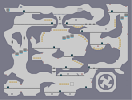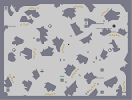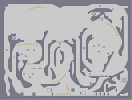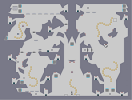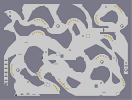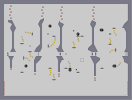The symbol Mining Ore Mining Copper Cave of Uncertainty Mining Iron They're not bunnies

Pages: (0)

### I didn't comment on this yet?

weird, I'm a huge Calvin & Hobbes fan. It's the only good newspaper comic ever. Seriously. Just recently, I looked back through my old Garfield comics and, well, lets just say it's back up on the shelf.

### awesome

looks great in full view as well. 5/5
Five!

5'd

### =D

Thanks.
PS - I just gave you your 1600th rating

### Yes!

My 5 got it up to a 3!

5

I <3 c&h

### ...

what? pakwan is a fucking dick. I'm just giving a shot at the mininalism like Wizard2 -_- Iban, I thought you knew who I was!

### omfg

is pakwan back again?

YEEEESSS

### OH MAH GAWD

I forgot you were going to do this! Awesome!!! Thanks for finishing what I was too lazy to do :p

### I would invite the people who sniped this map to come forward.

Hiding in the shadows means you're a punk bitch. At least take credit for what you're doing.

-origami_alligator-

### PLUS...

the prequel, which is the reason why I chose Hobbes is mine only too. It felt right to use mines only in this one as well.

### sorry Njitsu

I have had bad experiences with black in the past in n-arts. The only other color would be orange, and the contrast with the red would make it look ugly. using something other than mines would be gold (hard to see) or triggers, which i dont like to use as stated above.

5aved

### well you've definitely proved you're good at drawing stuff

but maybe try filling it in with some color? or using anything other than mines?

### Thanks

<3
i like that it looks good in thumbnail and in full view. awesome n art. and i <3 hobbes :D

### thus

is of course awesome

<3

love that comic.
as in

awesome
YES